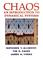### Introduction to Mathematical Chaos Theory for Engineers

#### Course Description

Fundamentals of stability theory and bifurcation. The Feigenbaum diagram. Universality principle, Schwarzian derivative. Periodic points, Sarkovski theorem. Sensitivity, the Ljapunov exponent. Fractals and fractal dimensions. Periodic points. Period 3 implies chaos. Chaotic one-hump iterators. Chaotic iterators on metric spaces. L- systems. Fluid convexity, the Lorenz model. Control of chaos.

#### General Competencies

General knowledge on the history of the Mathematical theory of chaos. Mathematical calculation of basic elements of Mathematical theory of chaos: fixed points, stability, periodic points, bifurcation, sensitive dependence on intial conditions. Basic knowledge on fractal geometry: mathematical generation of fractals, self similarities, fractal dimensions. Basic criteria for chaotic behaviour of continuous iterators.

#### Learning Outcomes

1. describe fundamental properties which characterize chaotic iterators
2. distinguish linear, nonlinear, nonchaotic and chaotic iteration processes
3. apply the Ljapunov method for estimating of sensitivity of processes
4. explain the importance of fractal sets and geometry in describing of chaotic iterators
5. manipulate with calculus of the theory of stability: stability of fixed points
6. manipulate with calculus of the theory of bifurcation and chaos: periods 2 and 3
7. analyze all four states on the path to chaos for continuous iterators
8. create an own chaotic circuit from basic elements purchased in Chipoteka

#### Forms of Teaching

Lectures

Based on mathematical skills and knowledge from first year of study, some new knowledge are adopted from mathematical theory on chatocal interators; computer simulation and visualization of fractal sets; basic knowledge on the fractal geometry; calculation of chatical properties; visualization of chaos on computer.

Exams

Oral exam: students continuously can solve and present their homework; there exists a final exam for advanced students. Written exams: control test and fianl exam; seminar work.

Consultations

By appointment.

Seminars

Seminar work is free choice, but it is appropriate for higher estimates. By seminar, a student shows: creativity, independence and the level of adopted knowledge.

Acquisition of Skills

Recognition of complex systems and process in nature, measurement of chatoc properties, work with fractal sets, introducing of new aspects of thinking.

Continuous Assessment Exam
Class participation 0 % 10 % 0 % 0 %
Seminar/Project 0 % 30 % 0 % 30 %
Mid Term Exam: Written 0 % 30 % 0 %
Final Exam: Written 0 % 30 %
Exam: Written 0 % 70 %

#### Week by Week Schedule

1. Reading of the first part of famous Gleick book on Chaos, an introduction for creativ way of thinkining, a computer demonstration on the existence of Chaos in the nature: 'vodenično kolo', mathematical and magnet pendulum, virbration, Lorentzov system
2. Continous iterator, fixed points, stability of fixed points, iterators as continous mapping from [0,1] into [0,1], domain of stability for one parametric iterations
3. Fractal sets which are appearing in the mathematical theory of chaos: Feigenbaum diagram and Cantor set
4. A continuation of the reading of Gleick book; the background on the one-hump iterator; the basis on the 'shift' iterator
5. Bifurcation of order 2, the cascade of bifucration, Feigenbaum number, the presentation of propositions for the working of a seminar from mathematical theory cahos
6. Mathematical generation of fractals: iteration functional system 'IFS' and the mathematical basis for Julija sets and Mandelbrot set
7. Sensitive dependence on initial conditions by definition, sensitive dependence on initial conditions for 'one-hump iterator' by using the Schwarzian derivative
8. Sensitive dependence on initial conditions by Ljapunov coefficient, universality of Chaos, the set 'Sigma 2', 'Sigma 2' as a metric space, sensitive dependence on initial conditions for 'Shift' iterator
9. Mathematical definition of Chaos: sensitive dependence on initial conditions, density of periodic points, transitivity of the set of periodic points
10. Composition of higher order, periodic points, Sarkovskii theorem, period 3 implies chaos
11. Computation of period 3 for continous iterators: critera for the existence and nonexistence of periodic points of order 3, methods for efective proving of period 3
12. Chaotic properties of 'one-hump iterators': theory and examples
13. Chaos in computer: chaotic behaviour of 'shift iterator'
14. Presentation and estimation of student seminar on Chaos
15. Presentation and estimation of student seminar on Chaos

#### Study Programmes

Computer Engineering (profile)
Mathematics and Science (1. semester)
Computer Science (profile)
Mathematics and Science (1. semester)
Control Engineering and Automation (profile)
Mathematics and Science (1. semester)
Electrical Engineering Systems and Technologies (profile)
Mathematics and Science (1. semester)
Electrical Power Engineering (profile)
Mathematics and Science (1. semester)
Electronic and Computer Engineering (profile)
Mathematics and Science (1. semester)
Electronics (profile)
Mathematics and Science (1. semester)
Information Processing (profile)
Mathematics and Science (1. semester)
Software Engineering and Information Systems (profile)
Mathematics and Science (1. semester)
Telecommunication and Informatics (profile)
Mathematics and Science (1. semester)
Wireless Technologies (profile)
Mathematics and Science (1. semester)

#### Literature

J. Banks, V. Dragan, A. Jones (2003.), Chaos. A mathematical introduction, Cambridge University Press
H.-O. Peitgen, H, Jurgens, D. Saupe (1992.), Chaos and Fractals. New Frontiers of Science, Springer
R. L. Devaney (1990.), Chaos, Fractals, and Dynamics, Addison-Wesley
T. Kapitaniak (2000.), Chaos for Engineers. Theory, Applications and Control, SpringerK. T. Alligood, T. D. Sauer, J. A. Yorke (1996.), Chaos. An introduction to dynamical systems, Springer, New York

#### General

ID 34561
Winter semester
4 ECTS
L3 English Level
L1 e-Learning
45 Lectures
0 Exercises
0 Laboratory exercises
0 Project laboratory